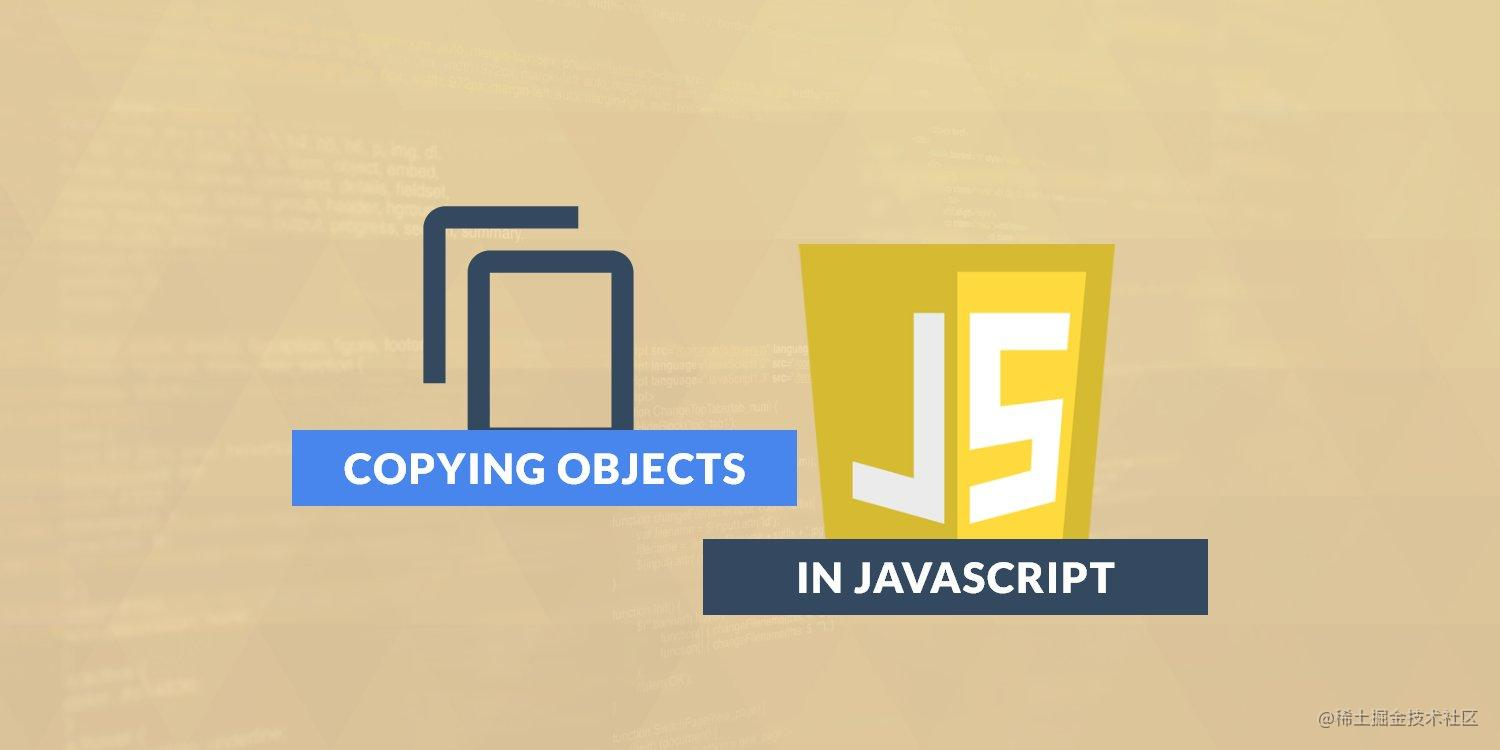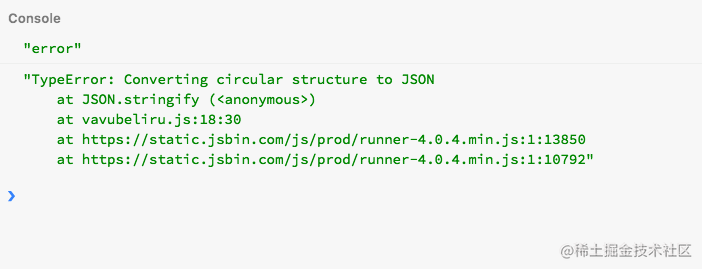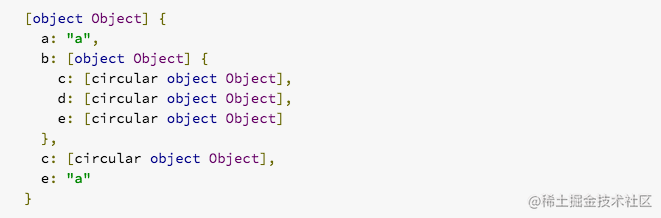# JavaScript 中的对象拷贝## 介绍

``````let obj = {
a: 1,
b: 2,
};
let copy = obj;

obj.a = 5;
console.log(copy.a);
// 结果
// copy.a = 5;``````

`obj` 变量是一个新对象初始化的容器。`copy` 变量指向同一个对象，是对该对象的引用。所以现在有两种方式可以访问这个 `{ a: 1, b: 2, }` 对象。你必须通过 `obj` 变量或 `copy` 变量，无论你是通过何种方式对这个对象进行的任何操作都会影响该对象。

## 复制对象的原始方式

``````function copy(mainObj) {
let objCopy = {}; // objCopy 将存储 mainObj 的副本
let key;

for (key in mainObj) {
objCopy[key] = mainObj[key]; // 将每个属性复制到objCopy对象
}
return objCopy;
}

const mainObj = {
a: 2,
b: 5,
c: {
x: 7,
y: 4,
},
}

console.log(copy(mainObj));``````

### 存在的问题

1. `objCopy` 对象具有一个新的 `Object.prototype`方法，这与 `mainObj` 对象的原型方法不同，这不是我们想要的。我们需要精确的拷贝原始对象。
2. 属性描述符不能被复制。值为 `false` 的 “可写(`writable`)” 描述符在 `objCopy` 对象中为 `true`
3. 上面的代码只复制了 `mainObj` 的可枚举属性。
4. 如果原始对象中的一个属性本身就是一个对象，那么副本和原始对象之间将共享这个对象，从而使其各自的属性指向同一个对象。

——— 愚人码头注，开始 ——

`writable` 设置为`false`时，表示不可写，也就是说属性不能被修改。

``````var o = {}; // Creates a new object

Object.defineProperty(o, 'a', {
value: 37,
writable: false
});

console.log(o.a); // logs 37
o.a = 25; // No error thrown
// (it would throw in strict mode,
// even if the value had been the same)
console.log(o.a); // logs 37. The assignment didn't work.

// strict mode
(function() {
'use strict';
var o = {};
Object.defineProperty(o, 'b', {
value: 2,
writable: false
});
o.b = 3; // throws TypeError: "b" is read-only
return o.b; // returns 2 without the line above
}());``````

——— 愚人码头注，开始 ——

## 浅拷贝对象

### 使用 Object.assign() 方法

Object.assign() 方法用于将从一个或多个源对象中的所有可枚举的属性值复制到目标对象。

``````let obj = {
a: 1,
b: 2,
};
let objCopy = Object.assign({}, obj);
console.log(objCopy);
// Result - { a: 1, b: 2 }``````

``````let obj = {
a: 1,
b: 2,
};
let objCopy = Object.assign({}, obj);

console.log(objCopy); // result - { a: 1, b: 2 }
objCopy.b = 89;
console.log(objCopy); // result - { a: 1, b: 89 }
console.log(obj); // result - { a: 1, b: 2 }``````

### Object.assign()的陷阱

``````let obj = {
a: 1,
b: {
c: 2,
},
}
let newObj = Object.assign({}, obj);
console.log(newObj); // { a: 1, b: { c: 2} }

obj.a = 10;
console.log(obj); // { a: 10, b: { c: 2} }
console.log(newObj); // { a: 1, b: { c: 2} }

newObj.a = 20;
console.log(obj); // { a: 10, b: { c: 2} }
console.log(newObj); // { a: 20, b: { c: 2} }

newObj.b.c = 30;
console.log(obj); // { a: 10, b: { c: 30} }
console.log(newObj); // { a: 20, b: { c: 30} }

// 注意: newObj.b.c = 30; 为什么呢..``````

#### obj.b.c = 30 ?

``````let someObj = {
a: 2,
}

let obj = Object.create(someObj, {
b: {
value: 2,
},
c: {
value: 3,
enumerable: true,
},
});

let objCopy = Object.assign({}, obj);
console.log(objCopy); // { c: 3 }``````

`someObj` 是在 `obj` 的原型链，所以它不会被复制。

## 深度拷贝对象

### 使用 JSON.parse(JSON.stringify(object));

``````let obj = {
a: 1,
b: {
c: 2,
},
}

let newObj = JSON.parse(JSON.stringify(obj));

obj.b.c = 20;
console.log(obj); // { a: 1, b: { c: 20 } }
console.log(newObj); // { a: 1, b: { c: 2 } } (一个新的对象)``````

## 复制对象方法

``````let obj = {
name: 'scotch.io',
exec: function exec() {
return true;
},
}

let method1 = Object.assign({}, obj);
let method2 = JSON.parse(JSON.stringify(obj));

console.log(method1); //Object.assign({}, obj)
/* result
{
exec: function exec() {
return true;
},
name: "scotch.io"
}
*/

console.log(method2); // JSON.parse(JSON.stringify(obj))
/* result
{
name: "scotch.io"
}
*/``````

## 复制循环引用对象

### 使用 JSON.parse(JSON.stringify(object))

``````// 循环引用对象
let obj = {
a: 'a',
b: {
c: 'c',
d: 'd',
},
}

obj.c = obj.b;
obj.e = obj.a;
obj.b.c = obj.c;
obj.b.d = obj.b;
obj.b.e = obj.b.c;

let newObj = JSON.parse(JSON.stringify(obj));

console.log(newObj); ``````### 使用 Object.assign()

``````// 循环引用对象
let obj = {
a: 'a',
b: {
c: 'c',
d: 'd',
},
}

obj.c = obj.b;
obj.e = obj.a;
obj.b.c = obj.c;
obj.b.d = obj.b;
obj.b.e = obj.b.c;

let newObj2 = Object.assign({}, obj);

console.log(newObj2); ```````Object.assign()` 适用于浅拷贝循环引用对象，但不适用于深度拷贝。随意浏览浏览器控制台上的循环引用对象树。我相信你会发现很多有趣的工作在那里。

## 使用展开操作符(…)

ES6已经有了用于数组解构赋值的 rest 元素，和实现的数组字面展开的操作符。看一看这里的数组的展开操作符的实现：

``````const array = [
"a",
"c",
"d", {
four: 4
},
];
const newArray = [...array];
console.log(newArray);
// 结果
// ["a", "c", "d", { four: 4 }]``````

``````let obj = {
one: 1,
two: 2,
}

let newObj = { ...z };

// { one: 1, two: 2 }``````